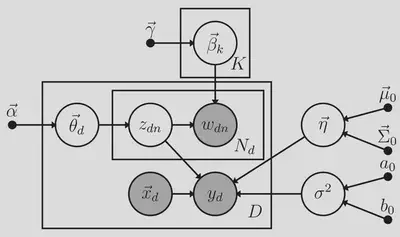# Supervised latent Dirichlet allocation with covariates: A Bayesian structural and measurement model of text and covariates

### Abstract

Text is a burgeoning data source for psychological researchers, but little methodological research has focused on adapting popular modeling approaches for text to the context of psychological research. One popular measurement model for text, topic modeling, uses a latent mixture model to represent topics underlying a body of documents. Recently, psychologists have studied relationships between these topics and other psychological measures by using estimates of the topics as regression predictors along with other manifest variables. While similar two-stage approaches involving estimated latent variables are known to yield biased estimates and incorrect standard errors, two-stage topic modeling approaches have received limited statistical study and, as we show, are subject to the same problems. To address these problems, we proposed a novel statistical model — supervised latent Dirichlet allocation with covariates (SLDAX) — that jointly incorporates a latent variable measurement model of text and a structural regression model to allow the latent topics and other manifest variables to serve as predictors of an outcome. Using a simulation study with data characteristics consistent with psychological text data, we found that SLDAX estimates were generally more accurate and more efficient. To illustrate the application of SLDAX and a two-stage approach, we provide an empirical clinical application to compare the application of both the two-stage and SLDAX approaches. Finally, we implemented the SLDAX model in an open-source R package to facilitate its use and further study.

Publication
PsyArXivDirected acyclic graph of the SLDAX model. Observed variables are represented by shaded circles: $w_{dn}$ denotes the $n$th word in document $d$; for subject $d$, $\vec{x}d$ denotes $p$ predictor scores and $y_d$ denotes the outcome for subject $d$. Latent variables are represented by unshaded circles: $z{dn}$ denotes topic assignments for each word in each document; $\vec{\theta}_d$ denotes the $K$ topic proportions for each document; $\vec{\beta}_k$ denotes the $V$ topic-word probabilities for topic $k$; $\vec{\eta}$ denotes the regression coefficients relating $\vec{x}_d$ and $\vec{\bar{z}}_d$ to $y_d$; $\sigma^2$ denotes the residual variance of $Y$. Fixed parameters are represented by dots: $\vec{\alpha}$ denotes the hyperparameters of the topic probabilities; $\vec{\gamma}$ denotes the hyperparameters of the topic-word probabilities; $\vec{\mu}_0$ and $\vec{\Sigma}_0$ denote the prior mean vector and covariance matrix of $\vec{\eta}$, respectively; $a_0$ and $b_0$ are the shape and rate hyperparameters for $\sigma^2$. A set of (conditionally) independent replicates (i.e., words given topics; documents; word probabilities given a topic) is represented by a rectangle.##### Kenneth Tyler Wilcox
###### Postdoctoral Associate

My research interests include Bayesian statistics, multilevel modeling, integrative data analysis, meta-analysis, topic modeling, mixture modeling, statistical programming, and cognition and clinical psychology.